# Gates/filters in Flow Cytometry Data Visualization

## abstract

The flowViz package provides tools for visualization of flow cytometry data. This document describes the support for visualizing gates (a.k.a. filters).

## Introduction

Tools to read in and otherwise manipulate flow cytometry data are implemented in the flowCore package. The flowViz package provides visualization support for such data. In this document we give examples dealing with gated or filtered data. Please consult the online documentation for more details on the high-level visualization functions used here.

## Filters and filter results

The flowCore package defines the concepts of `filters` and `filterResults`. Filters are abstract entities defined in terms of markers that are measured on the flow instrument as different channels, whereas a filter result is the result of applying a filter to one or more `flowFrames` (data objects representing individual FCM experiments). Some filters are data driven, while some are not. We will later see how this distinction has an impact on their plotting. All abstractions of filters or gates inherit from class `filter`, whereas objects generated as the result of applying a filter inherit from class `filterResult`.

## Visualization

Before we discuss how to visualize gates in flowViz, we need to point out that this may only be reasonable for certain types of the many possible plots, namely one-dimensional density plots and two-dimensional scatter plots. Over time we might add gate-plotting support for other plot types as well. There are two principal ways to add gates to a flow cytometry plot; either we render the outlines of the gate region (for both one and two-dimensional plots), or we highlight the points within a gate region by distinctive color, glyphs, or point size (this applies for two-dimensional plots only). It only makes sense to visualize the boundaries of a filter if it has a (one or two dimensional) geometric representation. This is true for rectangle, ellipsoid and polygon gates, which are all frequently used. It is also true for some data driven gates, e.g. `norm2Filter` gates, which have a (data dependent) spherical representation. Also, it makes sense to draw gate boundaries only when plotting (some of) the channels that define the gate.

Visualizing `filterResults` is more general. Specifically, the result of applying a filter is usually a logical (`TRUE` or `FALSE`) vector for each cell, or more generally, a `factor` (as long as we restrict ourselves to non-fuzzy filters). This can be used as a grouping variable within a display, also when plotting channels other than those defining the gate.

All data-driven filters depend on the `filterResult` to be computed in order to plot them. The user doesn't have to worry about this fact, as the software will implicitly compute these objects if necessary. However, realizing filters and creating `filterResult` is often computationally intense and time-consuming, and in many cases makes sense to explicitly create the `filterResult` once and pass it on to the plotting functions instead of the input filter. In the course of this manual, `filter` objects and `filterResults` may be used interchangeably, unless stated otherwise.

## Example Data

We use the `GvHD` data set to provide some examples. It come as a serialized `flowSet` with the flowViz package and the interested user is referred to its documentation for details. For the purpose of this demonstration it is sufficient to know that the `phenoData` slot of the `GvHD` set contains several factor variables, `Patient` and `Visit` two of the most descriptive ones. In general, all `flowSets` contain an implicit `name` variable which is is used as the default conditioning variable in all of flowViz's high-level plotting functions.

``````library(flowViz)
data(GvHD)
head(pData(GvHD))
``````
``````##       Patient Visit Days Grade  name
## s5a01       5     1   -6     3 s5a01
## s5a02       5     2    0     3 s5a02
## s5a03       5     3    6     3 s5a03
## s5a04       5     4   12     3 s5a04
## s5a05       5     5   19     3 s5a05
## s5a06       5     6   26     3 s5a06
``````

The set is quite large and reducing it to a reasonable subset will speed up things for our interactive demonstration purpose. Due to various constrains (data size, complexity of computations, limitations in the grid software underlying the lattice and flowViz packages), rendering plots is usually not instantaneous. It usually takes a little bit of time to output complex panel layouts. When producing postscript output, the user should be aware that file size may become an issue, and we will address solutions for this problem at the end of this document.

The lattice package offers inline subsetting capabilities for all high level plotting functions though the `subset` argument, and this functionality (like most of the other typical lattice concepts) is also available for all derived plotting methods in flowCore. The general idea here is that all symbols defined in either the formula or one of the special arguments like `subset` are evaluated in the context of the `flowFrame's` `phenoData` data frame or the raw data matrix. This is a slight extension of the fundamental lattice idea necessitated by the fact that raw data and annotation data are stored separately in a flowSet.

``````xyplot(`SSC-H` ~ `FSC-H` | Visit, data=GvHD,
subset=Patient=="6")
``````

Inline subsetting is useful to change plots on the fly, or to play around with various panel arrangements. However, we still pass the whole `GvHD` object down to the plotting function, potentially increasing memory usage and decreasing performance due to unnecessary heavy copying operations. Since we only want to use the subset for patient 6 in the following examples, it makes sense to directly subset the `flowSet`.

``````GvHD <- GvHD[pData(GvHD)\$Patient==6]
``````

We also want to transform some of the fluorescence channels to an adequate log-like scale. A good choice is the `asinh` function which can deal with negative values.

``````tf <- transformList(from=colnames(GvHD)[3:7], tfun=asinh)
GvHD <- tf %on% GvHD
``````

For details on this step, please see the documentation of the `transformList` and `\%on\%` functions in the flowCore package.

## Filters in scatter plots

Trellis scatter plots are created using `xyplot` methods. The `xyplot` method for `flowSet` objects supports filters through the `filter` argument. As mentioned before, its value can either be an object inheriting from class `filter` or a `filterResult` (a `filterResultList` of multiple `filterResults` for `flowSets`).

The key concepts here are that

• `filters` and `filterResults` can be used interchangeably. Providing `filterResults` directly may increase performance for data-driven filters.

• visualization of `filters` depends on the type of rendering. For example, with `smooth=TRUE`, filters are visualized geometrically, which makes sense only under certain circumstances (e.g. display axes matching filter parameters, the `filter` class has a geometric represention). For scatter plots of individual dots for all events (`smooth=FALSE`), filters are visualized through grouping and/or geometric filter outlines; the former makes sense more generally, as display variables need not match filter parameters. Grouping has the drawback of overplotting. The effect of overplotting can be reduced somewhat using transparency, but scalability issues remain. We may try dealing with this at some point if it becomes enough of a hassle.

• the software will check for matching parameters and available visualization representations as much as possible, yet finally it is up to the user to construct reasonable calls to the plotting functions.

## Simple Geometric Filter Types

Visualization of most of the typical FCM filter types is straight forward and doesn't require the computation of `filterResult`. All parameters (e.g. min and max values in a recangular gate) are unambigously defined in the `filter` object. We will start with a simple `rectangleGate` in the `FSC-H` and `SSC-H` dimensions.

As default for smoothed scatter plots, the gate boundaries are drawn. Orientation of the gate will be handled by the plotting function and the user doesn't have to worry about the order of arguments when defining the `filter`. The software also decides on a reasonable two-dimensional geometric representation of more complex filters. E.g., we can add a third dimension to the `rectangleGate` and still achive the same visualization in the `FSC-H` and `SSC-H` dimensions.

``````rgate2 <- rgate * rectangleGate("FL1-H"=c(2, 4))
xyplot(`SSC-H` ~ `FSC-H` | Visit, data=GvHD, filter=rgate2)
``````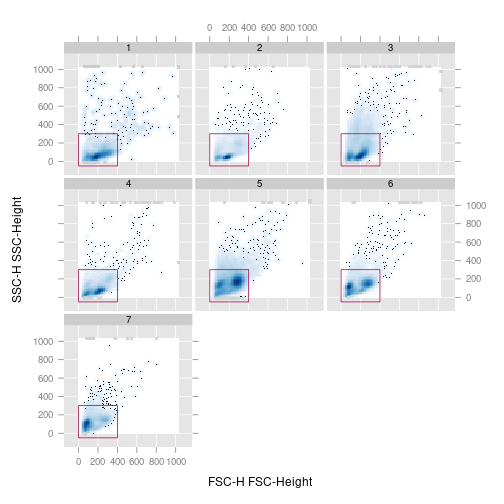Most of the customization options defined in the lattice package will also work here. A more detailed discussion about the filter-specific graphical settings will follow in one of the later sections.

For the non-smoothed rendering (i.e., all events are plotted as individual points).

``````xyplot(`SSC-H` ~ `FSC-H` | Visit, data=GvHD, filter=rgate, smooth=FALSE)
``````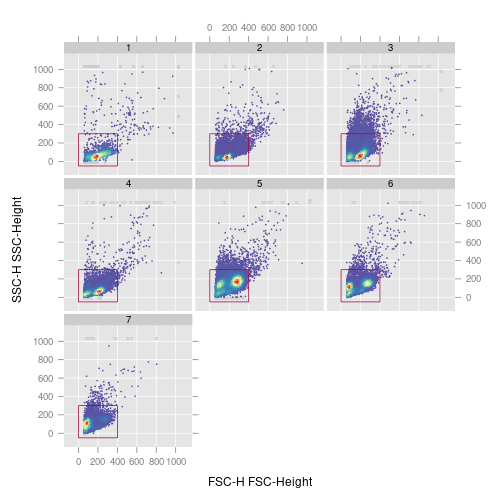Sometime non-smoothed plots can be slow for large number of events. We provide hexbin version of xyplot for faster rendering.

``````xyplot(`SSC-H` ~ `FSC-H` | Visit, data=GvHD, filter=rgate, smooth=FALSE,xbin=128)
``````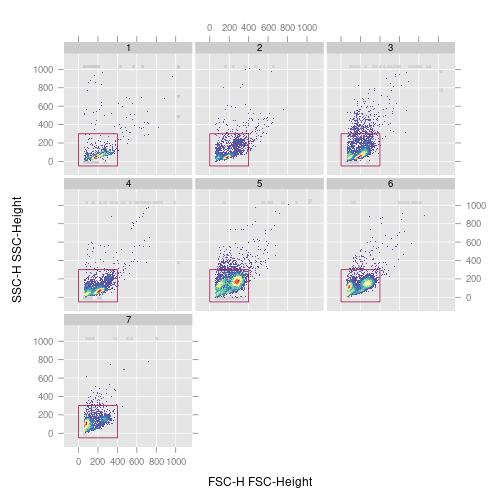Additionally, We can add statistics of gated population along with the `filter` to the plot using the `stat` argument.`pos` and `abs`(whether the position is absolute or relative to filter boundary ) are used to control the label position.

``````xyplot(`SSC-H` ~ `FSC-H` | Visit, data=GvHD, filter=rgate,
smooth=FALSE, stat=TRUE,pos=0.5,abs=TRUE)
``````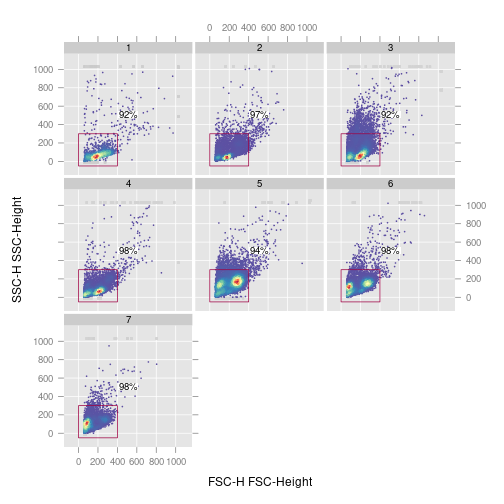Sometime it is necessary to display multipe filters/gates in one panel to represent multiple sub-populations gated from the same parent populatuion.

``````recGate1<-rectangleGate("FL4-H"=c(3.3,5.3),"FL2-H"=c(2.5,5))
recGate2<-rectangleGate("FL4-H"=c(4,6),"FL2-H"=c(6,8))
filters1<-filters(list(recGate1,recGate2))
xyplot(`FL2-H` ~ `FL4-H`
,data=GvHD[]
,filter=filters1
,stat=TRUE
,margin=FALSE
)
``````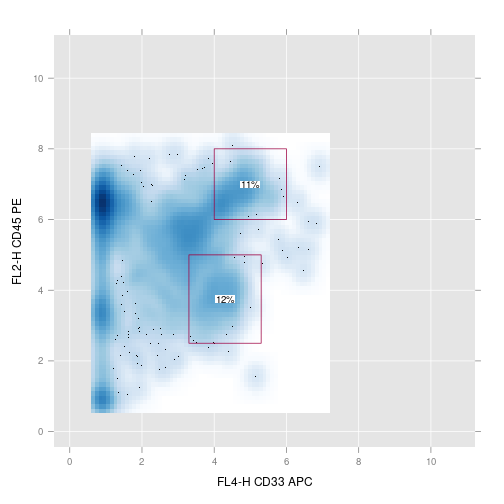The constraint for a valid two-dimensional representation doesn't apply any more for this type of rendering. Since each event is represented by a single point on the plot, we can visualize arbitrarily complex `filters` using different colors or point glyphs for the different sub-populations. However this implies that we need to evaluate each filter, i.e., we need to figure out which of the events are in and which are outside of the filter. We will see in a minute how we can optimize these operations. Going back to our three-dimensional rectangle, we notice that not all points within the rectangle are also color red, because the additional third dimension further reduced the selection of positive events in the `FSC-H` and `SSC-H` dimensions.

``````xyplot(`SSC-H` ~ `FSC-H` | Visit, data=GvHD, filter=rgate2,
smooth=FALSE, outline=TRUE)
``````%

Overplotting is a potential problem for this representation. As a matter of fact, it is problematic for all visualization approaches of high-volume FCM data that try to display individual events rather than some form of summary statistic like density estimates. Partial transparency can alliviate some of these shortcomings but this approach does not scale well and has certain technical limitations on graphic devices that don't support transparency. We will see in one of the following sections how to enable partial transparency in scatter plots.

## Data-Driven Filters

Data-driven filters don't have a natural geometric representation a priori; they are data-driven. Once all parameters are estimated, we can usually derive such a representation from the `filterResult`. There is only a small subet of `filter` classes for which this is not directly possible (`kmeansFilter`, `timeFilter`), although one might be able to fall back to approximations like convex hulls or similar. So far, flowViz has nor support for plotting gate outlines of these filter types, and they are silently ignored when passed on as argument `filter` to any of the high-level plotting functions. As shown in the previous example, the absence of a two-dimensional spherical representation only poses a problem when trying to plot filter boundaries, the non-smooth representation of individual events is available for virtually all `filter` types.

In the following example, we first compute the `filterResults` of a `norm2Filter` in `FSC-H` and `SSC-H` for each frame in our `GvHD` `flowSet` and plot their geometric elliptic representation.

``````n2Filter <- norm2Filter("SSC-H", "FSC-H", scale=2, filterId="Lymphocytes")
n2Filter.results <- filter(GvHD, n2Filter)
xyplot(`SSC-H` ~ `FSC-H` | Visit, data=GvHD, filter=n2Filter.results)
``````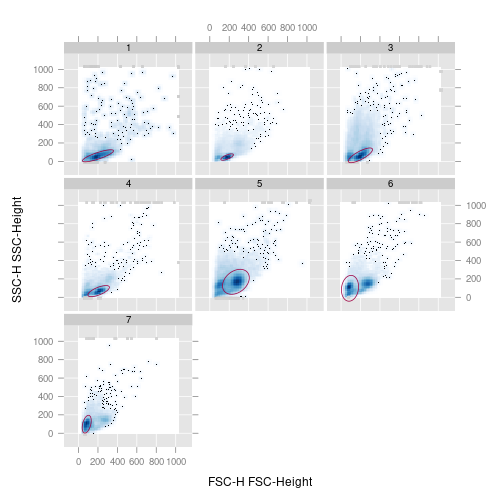%

There are data-driven filter types that may result in more than just one sub-population. `curv1Filters` and `curv2Filters` are a prominent example. In the next code chunk we use a `curv2Filter` to identify high-density areas in the `FSC-H` and `FL4-H` projections of the data.

``````library(flowStats)
c2f <- curv2Filter("FSC-H", "FL4-H", bwFac=1.8)
c2f.results <- filter(GvHD, c2f)
xyplot(`FL4-H` ~ `FSC-H` | Visit, data=GvHD, filter=c2f.results)
``````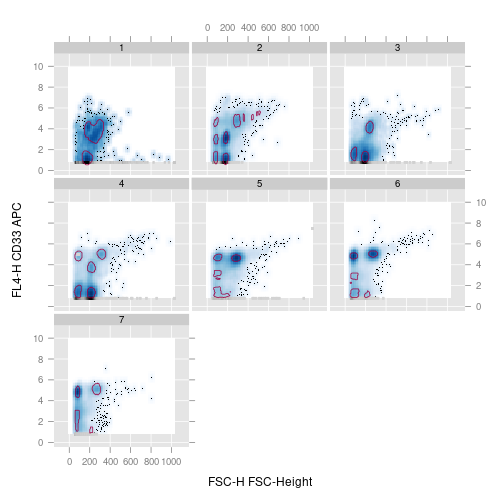%

This is a good example for a case where passing the `filterResult` instead of the `filter` helps to optimize computation time. Depending on the hardware it can take quite a while to complete the extensive computations necessary for the identification of siginficant high-density areas in two dimensions. Imagine you want to make slight changes to your plot (and producing good graphical output is almost always an iterative approach); having to recompute the filterResult on each iteration would be very time-cosuming and annoying at best.

## Filters in one-dimensional density plots

The lattice package provides the high-level `densityplot` function to draw one-dimensional Kernel density estimates of univariate data. Density estimates are a generalization of the well-known histograms; instead of drawing boxes of relative or absolute frequencies of binned observations, a continous relative density is displayed, usually beautified by applying a smoother function with an appropriate bandwidth. The `densityplot` methods in the flowViz package assume an implicit conditioning variable (the measurement channel or channels) and display the density estimates of all frames for one channel as a stacked layout. This has proven to be a useful visualization, since the direct comparison of univariate channel distributions is of great interest in many FCM application, however the typically large number of samples makes superimposing in a single display impratical. See the documention of `densityplot` for more details.

In one-dimensional density plots it only makes sense to plot filter boundaries. The densities are basically a summary of the distribution of events for a particular channel, and there is no real notion of individual events any more. Similar to the `xyplot` methods, we can pass `filters` or `filterResults` to the function as optional argument `filter`. We decided to indicate gate boundaries by different shading of the the respective integrals of the density regions rather than simply adding vertical lines. These tend to be distracting in the stacked layout or are often partly masked by overplotting of neighbouring density areas.

``````densityplot(~ `FSC-H`, GvHD, filter=curv1Filter("FSC-H"))
``````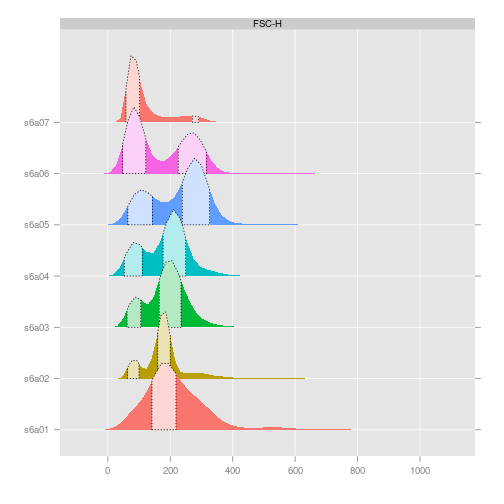The situation gets slightly more complex when we are conditioning on multiple FCM channels. In this case, filter has to be a list of equal length as the number of channels that are supposed to be displayed (i.e., the number of panels). Each list item may contain one of the familiar objects of class `filter`, `filterResult`, `filterResultList`, or `NULL` in case no filter should be plotted at all for a given panel.

``````densityplot(~ ., GvHD, channels=c("FSC-H", "SSC-H", "FL1-H"),
filter=list(curv1Filter("FSC-H"), NULL, rgate2))
``````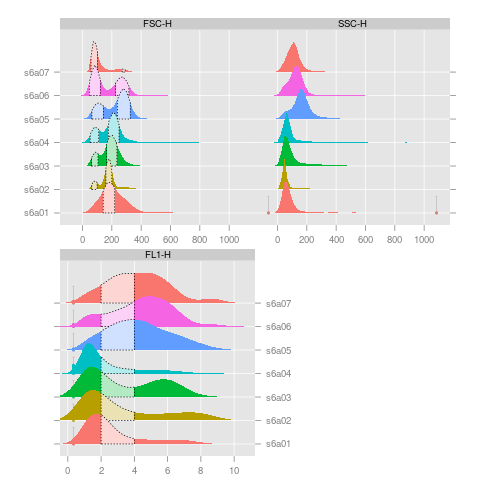%

Note that by default the scale of the x axis has been adjusted for each panel. This is contrary to the default settings in the lattice package and can be reversed using appropriate settings in in the `scales` argument.

## Plotting parameters

Lattice graphics are a great tool to easily create informative and consise data visualization with a minimal amount of code. Most of its default graphical parameters are well thought through. However, there is also a wealth of customization available, and we refer readers to the package's documentation as well as Deepayan Sarkar's excellent book “Lattice: Multivariate Data Visualization with R” for details. With respect to flowViz, it should suffice to mention that customization follows the exact same principles defined in the lattice package. As a matter of fact, most customization actually happens at the level of the underlying lattice software. The facts to mention are:

• There are session-wide global defaults that can be queried and set using the `flowViz.par.get` and `flowViz.par.set` functions. These are extensions to the `trellis.par.get` and `trellis.par.set` functions in the lattice package, and work in exactly the same way. All graphical parameters that are not defined in flowViz are directly passed on to lattice.

• Parameters can also be set for a single function call using the `par.settings` argument. These setting take precedence over global settings.

• Parameters are split up into logical categories and have to be provided as named lists or lists of lists. The categories directly relevant for filter plotting are `gate`, `gate.density`, and `gate.text`, the basic flowViz settings are controlled by the `flow.symbol` category. See the documentation for `flowViz.par.set` for a complete list of available parameters. All additional graphical parameters (like `cex`, `pch` and `col`) known from the lattice high-level plotting functions are also still available.

``````xyplot(`SSC-H` ~ `FSC-H` | Visit, data=GvHD, filter=rgate,
par.settings=list(gate=list(fill="black", alpha=0.2)))
``````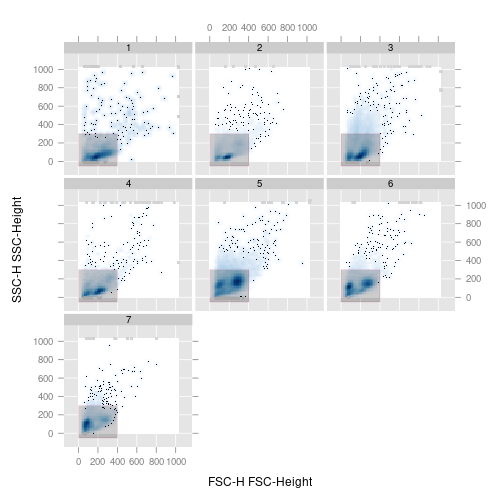%

We could have achieved the same customization by changing the session defaults:

``````flowViz.par.set(gate=list(fill="black", alpha=0.2))
xyplot(`SSC-H` ~ `FSC-H` | Visit, data=GvHD, filter=rgate)
``````

Depending on the rendering, the graphical parameters may have slightly different effects. The `col` parameters for instance sets the line color for filter boundaries and the point color for non-smooth scatter plots (and also the outline if `outline=TRUE`). In the following code sample we try to make use of partial transparency to address the problem of overplotting. Dense regions of the plot with many overplotted points will apprear darker. Although this is better than using opaque colors, lots of the underlying structure could still be hidden and we strongly emphasize the advantages of smoothed scatter plots for vizualization of FCM data.

``````xyplot(`SSC-H` ~ `FSC-H` | Visit, data=GvHD, filter=rgate,
smooth=FALSE,
par.settings=list(gate=list(col="orange", alpha=0.04,
pch=20, cex=0.7),
flow.symbol=list(alpha=0.04, pch=20, cex=0.7)))
``````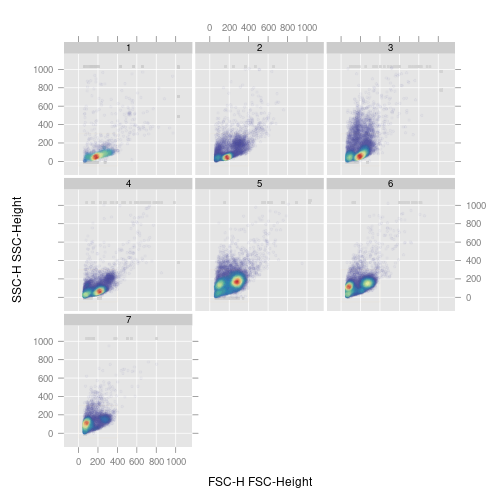For multiple population filters, R ordinary recycling rules apply, and we can spice up out plot of the `curv2Filter` results by using a different color for each sub-population.

``````xyplot(`FL4-H` ~ `FSC-H` | Visit, data=GvHD, filter=c2f.results,
par.settings=list(gate=list(fill=rainbow(10), alpha=0.5, col="transparent")))
``````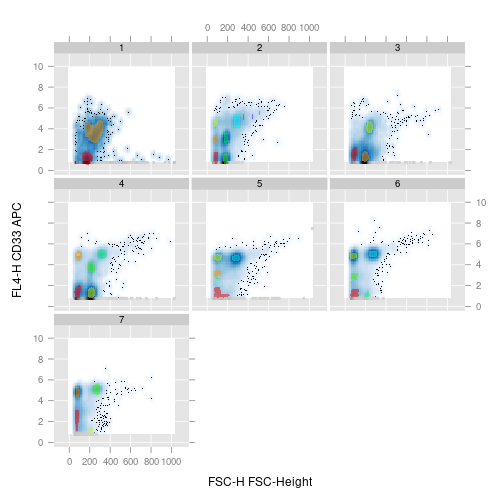Sometimes it helps to add population names to a plot. flowCore provides default names for each `filter` and their associated results, but the user is free to pass their own custom names. The interface is very simple: argument `names` either takes a logical scalar (default names are used if `TRUE`) or a character vector. Again, recycing rules apply when there are multiple sub-populations (unless default names are uses in which case the software provides a reasonable choice).

``````xyplot(`SSC-H` ~ `FSC-H` | Visit, data=GvHD, filter=n2Filter.results,
names=TRUE,
par.settings=list(gate=list(fill="black", alpha=0.2,
col="transparent"),
gate.text=list(col="darkred", alpha=0.7, cex=0.6)))
``````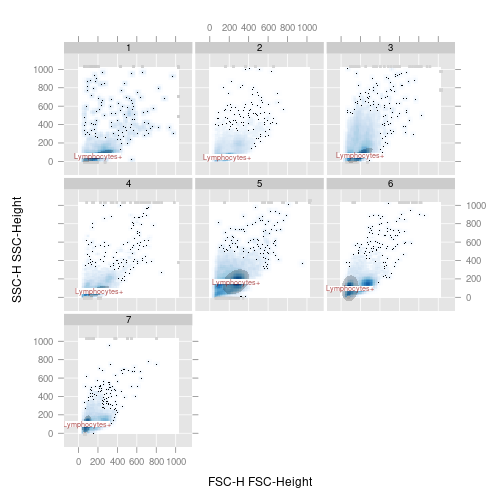## Restrictions on the formula interface

We mentioned before that all elements of the formulae used in flowViz are evaluated in the context of either the raw data matrix or the `phenoData` slot. This implies that formula components can themselves be valid R expressions including function calls. To a certain extend this is true, and the user might be able to produce reasonable graphical output by evaluating expressions on existing data or annotation objects, however we strongly discourage this use. The software makes certain assumptions on the relationship between gate definitions and data, the most prominent one being that both are on the same scale. The use of expressions in flowViz potentially changes the scale of the raw data, in which case the gate representation doesn't make much sense anymore. Furthermore, FCM data is naturally censored (by the available measurement range of the instrument) and we try to protect the user from irritating visual artifacts caused by the piling up of events on the margins of the data range. This is most notable in the one-dimensional densityplots, where densities are only computed within the data range, and margin events are added as vertical bars. Similarily, in the two-dimensional smoothed scatter plots, we display margin events as ticks around the actual data display. Again, changing the scale of the data would potentially invalidate many of the necessary computations.

In summary, lattice encourages the use of expressions in the formula interface and for the sake of customizability we didn't want to exclude this feature completely in the flowViz methods. However, the tool has to be used with great care, particularly when plotting gates.

## Filters in parallel coodinate plots

Although the `filter` argument does not make much sense except in scatter plots, `filterResults` can be used for grouping in other contexts. So far, only the `parallel` method supports this syntax.

``````parallel(~ . | Visit, GvHD, filter=n2Filter.results, alpha = 0.01)
``````